# Generative Models vs Discriminative Models: Which One to Choose?Machine learning (ML) and deep learning (DL) are two of the most exciting and constantly changing fields of study of the 21st century. Using these technologies, machines are given the ability to learn from past data and predict or make decisions from future, unseen data.

The inspiration comes from the human mind, how we use past experiences to help us make informed decisions in the present and the future. And while there are already many applications of ML and DL, the future possibilities are endless.

Computers utilize mathematics, algorithms, and data pipelines to draw meaningful inferences from raw data since they cannot perceive data and information like humans - not yet, at least. There are two ways we can improve a machine’s efficiency: either get more data or come up with newer or more robust algorithms.

Quintillions of data are generated all over the world almost daily, so getting fresh data is easy. But in order to work with this gigantic amount of data, we need new algorithms or we need to scale up existing ones.

Mathematics, especially branches like calculus, probability, statistics, etc., is the backbone of these algorithms or models. They can be widely divided into two groups:

1. Discriminative models
2. Generative models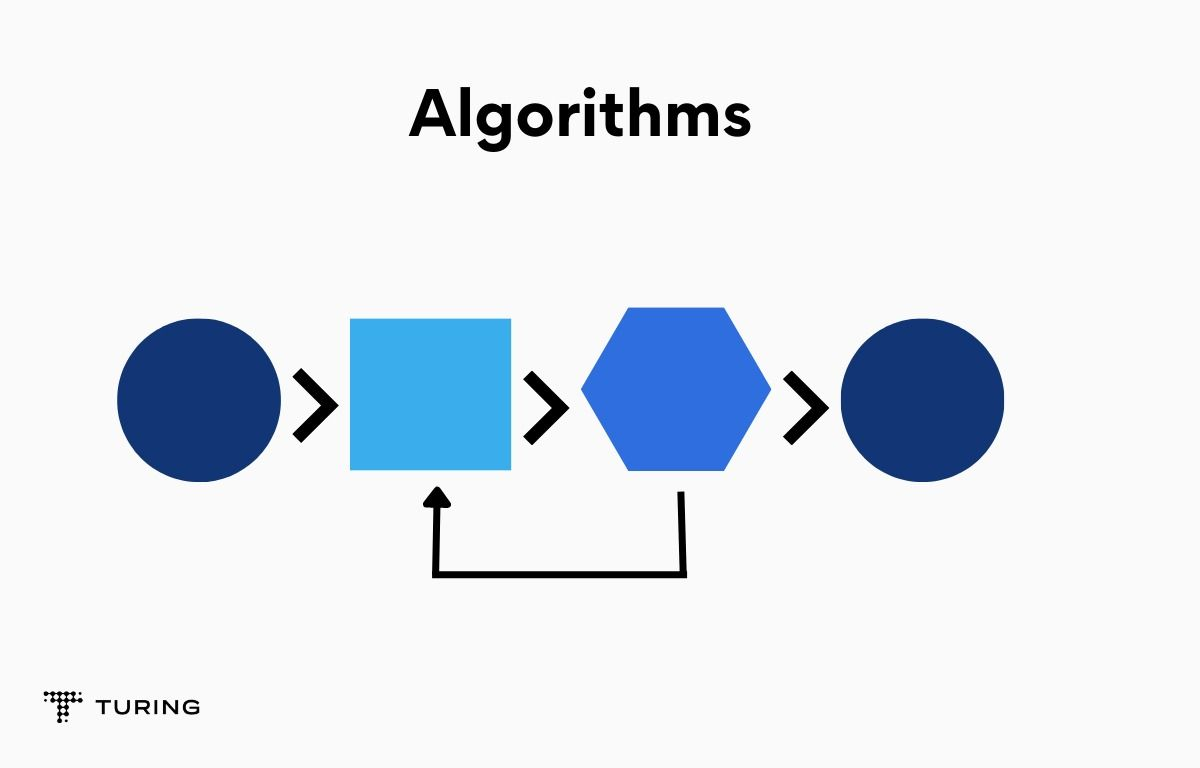This article will discuss both models. In order to understand them better, we begin with a short story.

## The topper twins

Zed and Zack are twin brothers. They’re so alike that you can’t tell who’s who by looking at them. The twins are child prodigies and jointly hold the topper’s position in their class. Zed can learn everything about a given topic. He goes in-depth and understands every little detail about a subject. Once he’s grasped it, he never forgets it. But, this is cumbersome, especially if there’s a lot to learn under said topic. What’s more, he has to prepare for his exams much sooner than his brother.

On the other hand, Zack studies by creating a mind map. He gets the general idea of a topic and then learns the differences and patterns between the subtopics. This gives him a lot more flexibility in his thinking process. You could say he learns by learning the differences.

As we can see, the brothers have very different learning approaches but both seem to work as is evident by the topper’s position they’ve held for so long.

Translating the analogy to our discussion, generative models work like Zed and discriminative models work like Zack.

Mathematically, generative classifiers assume a functional form for P(Y) and P(X|Y), then generate estimated parameters from the data and use the Bayes’ theorem to calculate P(Y|X) (posterior probability). Meanwhile, discriminative classifiers assume a functional form of P(Y|X) and estimate the parameters directly from the provided data.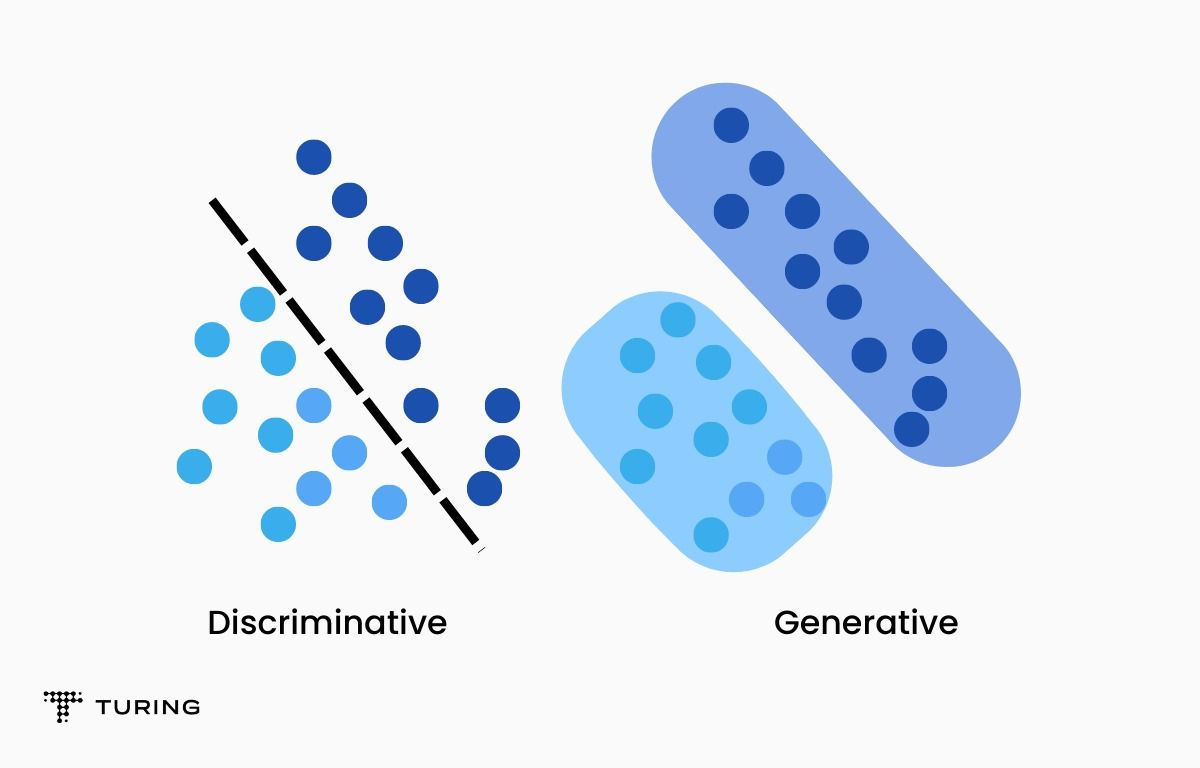## Discriminative model

The majority of discriminative models, aka conditional models, are used for supervised machine learning. They do what they ‘literally’ say, separating the data points into different classes and learning the boundaries using probability estimates and maximum likelihood.

Outliers have little to no effect on these models. They are a better choice than generative models, but this leads to misclassification problems which can be a major drawback.

Here are some examples and a brief description of the widely used discriminative models:

1. Logistic regression: Logistic regression can be considered the linear regression of classification models. The main idea behind both the algorithms is similar, but while linear regression is used for predicting a continuous dependent variable, logistic regression is used to differentiate between two or more classes.

2. Support vector machines: This is a powerful learning algorithm with applications in both regression and classification scenarios. An n-dimensional space containing the data points is divided into classes by decision boundaries using support vectors. The best boundary is called a hyperplane.

3. Decision trees: A graphical tree-like model is used to map decisions and their probable outcomes. It could be thought of as a robust version of If-else statements.

A few other examples are commonly-used neural nets, k-nearest neighbor (KNN), conditional random field (CRF), random forest, etc.

## Generative model

As the name suggests, generative models can be used to generate new data points. These models are usually used in unsupervised machine learning problems.

Generative models go in-depth to model the actual data distribution and learn the different data points, rather than model just the decision boundary between classes.

These models are prone to outliers, which is their only drawback when compared to discriminative models. The mathematics behind generative models is quite intuitive too. The method is not direct like in the case of discriminative models. To calculate P(Y|X), they first estimate the prior probability P(Y) and the likelihood probability P(X|Y) from the data provided.

Putting the values into Bayes’ theorem’s equation, we get an accurate value for P(Y|X).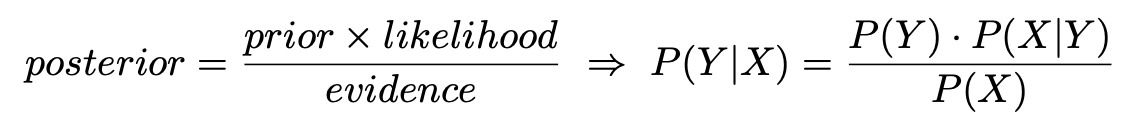Here are some examples as well as a description of generative models:

1. Bayesian network: Also known as Bayes’ network, this model uses a directed acyclic graph (DAG) to draw Bayesian inferences over a set of random variables to calculate probabilities. It has many applications like prediction, anomaly detection, time series prediction, etc.

2. Autoregressive model: Mainly used for time series modeling, it finds a correlation between past behaviors to predict future behaviors.

3. Generative adversarial network (GAN): It’s based on deep learning technology and uses two submodels. The generator model trains and generates new data points and the discriminative model classifies these ‘generated’ data points into real or fake.

Some other examples include Naive Bayes, Markov random field, hidden Markov model (HMM), latent Dirichlet allocation (LDA), etc.

## Discriminative vs generative: Which is the best fit for Deep Learning?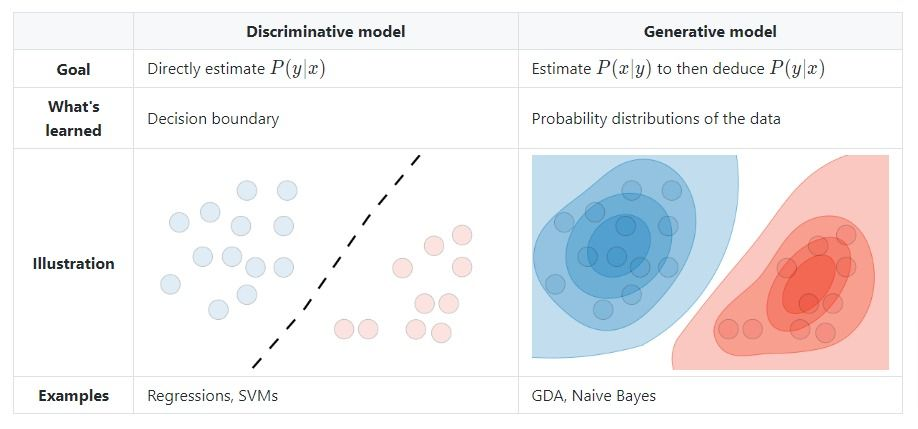Image source: Supervised learning cheatsheet

Discriminative models divide the data space into classes by learning the boundaries, whereas generative models understand how the data is embedded into the space. Both the approaches are widely different, which makes them suited for specific tasks.

Deep learning has mostly been using supervised machine learning algorithms like artificial neural networks (ANNs), convolutional neural networks (CNNs), and recurrent neural networks (RNNs). ANN is the earliest in the trio and leverages artificial neurons, backpropagation, weights, and biases to identify patterns based on the inputs. CNN is mostly used for image recognition and computer vision tasks. It works by pooling important features from an input image. RNN, which is the latest of the three, is used in advanced fields like natural language processing, handwriting recognition, time series analysis, etc.

These are the fields where discriminative models are effective and better used for deep learning as they work well for supervised tasks.

Apart from these, deep learning and neural nets can be used to cluster images based on similarities. Algorithms like autoencoder, Boltzmann machine, and self-organizing maps are popular unsupervised deep learning algorithms. They make use of generative models for tasks like exploratory data analysis (EDA) of high dimensional datasets, image denoising, image compression, anomaly detection and even generating new images.

This Person Does Not Exist - Random Face Generator is an interesting website that uses a type of generative model called StyleGAN to create realistic human faces, even though the people in these images don’t exist!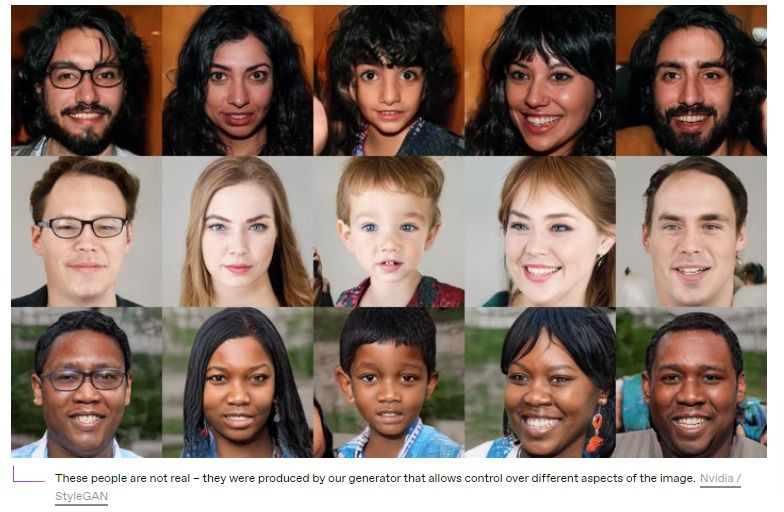Image source: This person does not exist' Creator reveals his Site's creepy origin story### Press

What's up with Turing? Get the latest news about us here.### Blog